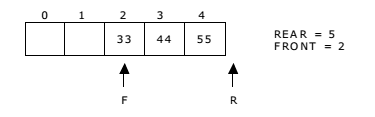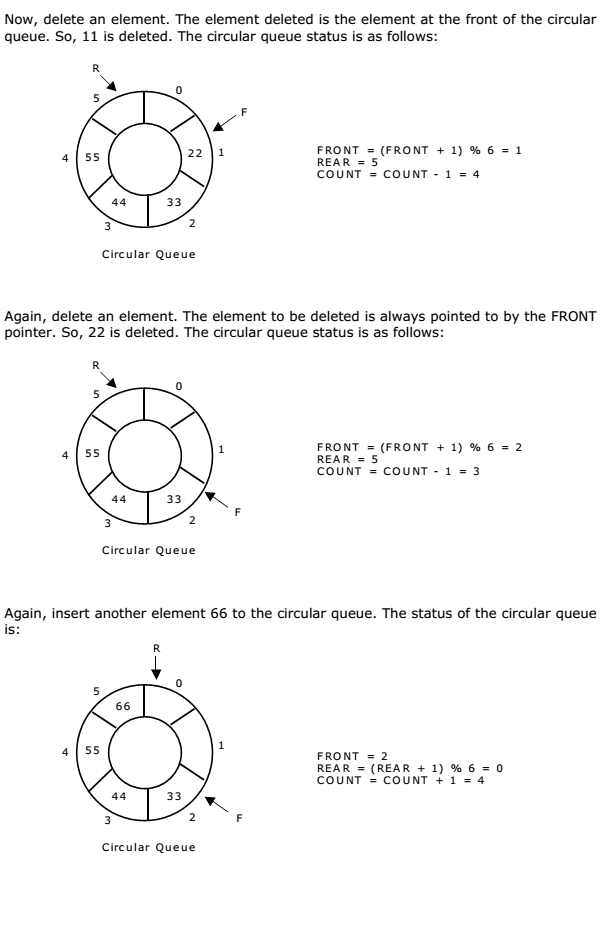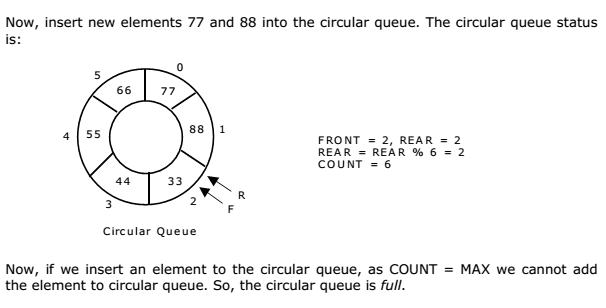A more efficient queue representation is obtained by regarding the array Q[MAX] as circular. Any number of items could be placed on the queue. This implementation of a queue is called a circular queue because it uses its storage array as if it were a circle instead of a linear list.

There are two problems associated with linear queue. They are: • Time consuming: linear time to be spent in shifting the elements to the beginning of the queue. • Signaling queue full: even if the queue is having vacant position.

For example, let us consider a linear queue status as follows:Next insert another element, say 66 to the queue. We cannot insert 66 to the queue as the rear crossed the maximum size of the queue (i.e., 5). There will be queue full signal. The queue status is as follows:This difficulty can be overcome if we treat queue position with index zero as a position that comes after position with index four then we treat the queue as a circular queue. In circular queue if we reach the end for inserting elements to it, it is possible to insert new elements if the slots at the beginning of the circular queue are empty.

Representation of Circular Queue:

Let us consider a circular queue, which can hold maximum (MAX) of six elements. Initially the queue is empty.You may be interested in:
Data Structures and Algorithms – MCQs.
Data Structures and Algorithms Online Tests